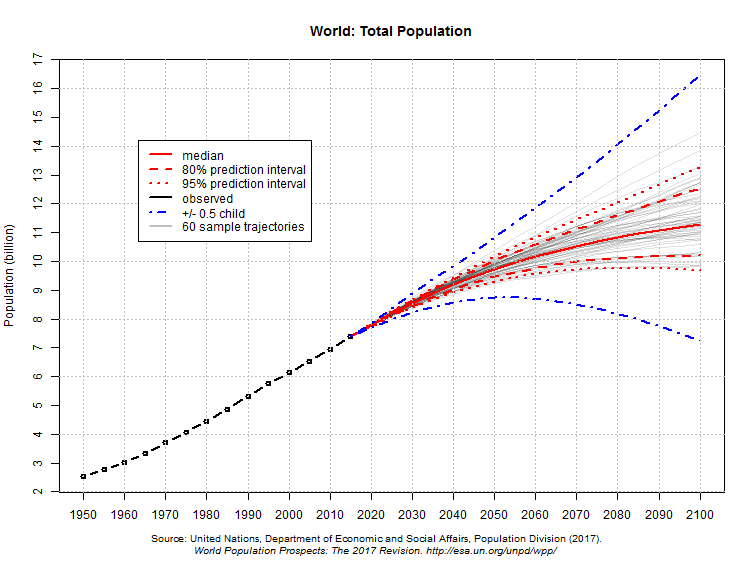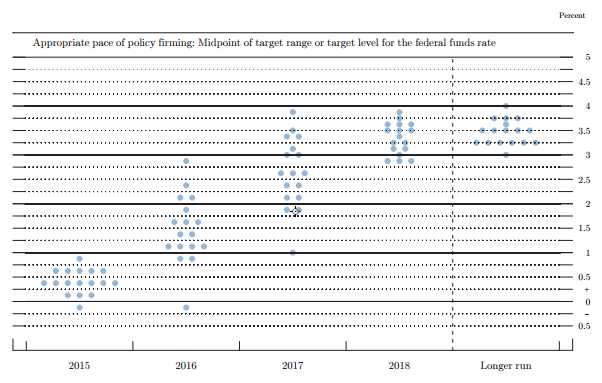## How to present subjective expert probability predictions to the public?

Another page discusses how to measure relative accuracy of expert probability forecasts in the context of estimating probabilities of future events. Consider now the context of estimating future numbers. For instance, suppose we ask different economists to estimate some economic statistic (inflation rate, unemployment rate, GDP growth, interest rate,etc) two years in the future. If we ask for a specific number, then displaying the different estimates as a histogram is natural, and retrospectively assessing an individual's accuracy over many such forecast questions can be done in essentially the same way as before, using MSE. But a sensible economist realizes the future is uncertain and that it is better to phrase predictions in terms of probabilities. Now a different problem immediately arises: how can we present these forecasts in some readily understandable way? In principle each expert has some probability distribution, but you can't really ask each of them to sketch a probability density function.

One solution is to combine the different predictions into a single probability distribution. The example below, from 2017, shows future world population projections obtained in that way.I want to contrast this with a second example. Since 2012, about four times a year the U.S. Federal Open Market Committee members make predictions about where the federal-funds rate will be at the ends of the next several years. These predictions are released in the form of "dot-plots" like the one below. Each dot represents one member's prediction for the end of each year. These dots were plotted in September 2015.But this is a truly bad way to request and display the information. The dots show each individual's median estimate; but it seems likely that the uncertainty in each individual estimate is as large as the variability amongst these medians. The "spread" of dots in the figure is liable to be mis-interpreted as showing the probability distribution for the federal-funds rate, whereas in fact the variability must be considerably larger than shown. And in fact it was 0.55 at the end of 2016, outside the range of dots.